Back to Contents

# Project 1: Pretty Pictures

So far we have been concerned only with programs which read and write text. But we have been sitting in front of a computer with graphical elements on the screen as well as textual ones.

There are many ways to produce pictures, both line drawing and photographic, using programming languages like Python. For this project, we will use the `turtle` module which uses a model of drawing invented for children but fun for adults too. In this model, there is a little ‘turtle’ on screen, and we direct it where to go, and it leaves a trail behind it as it goes.

To begin, we import the `turtle` module, and create a new turtle, which we call `t`:

``````Python
>>> import turtle
>>> t = turtle.Turtle()``````

Upon typing the second line, a blank window appears, with the turtle represented by an arrow, pointing to the right: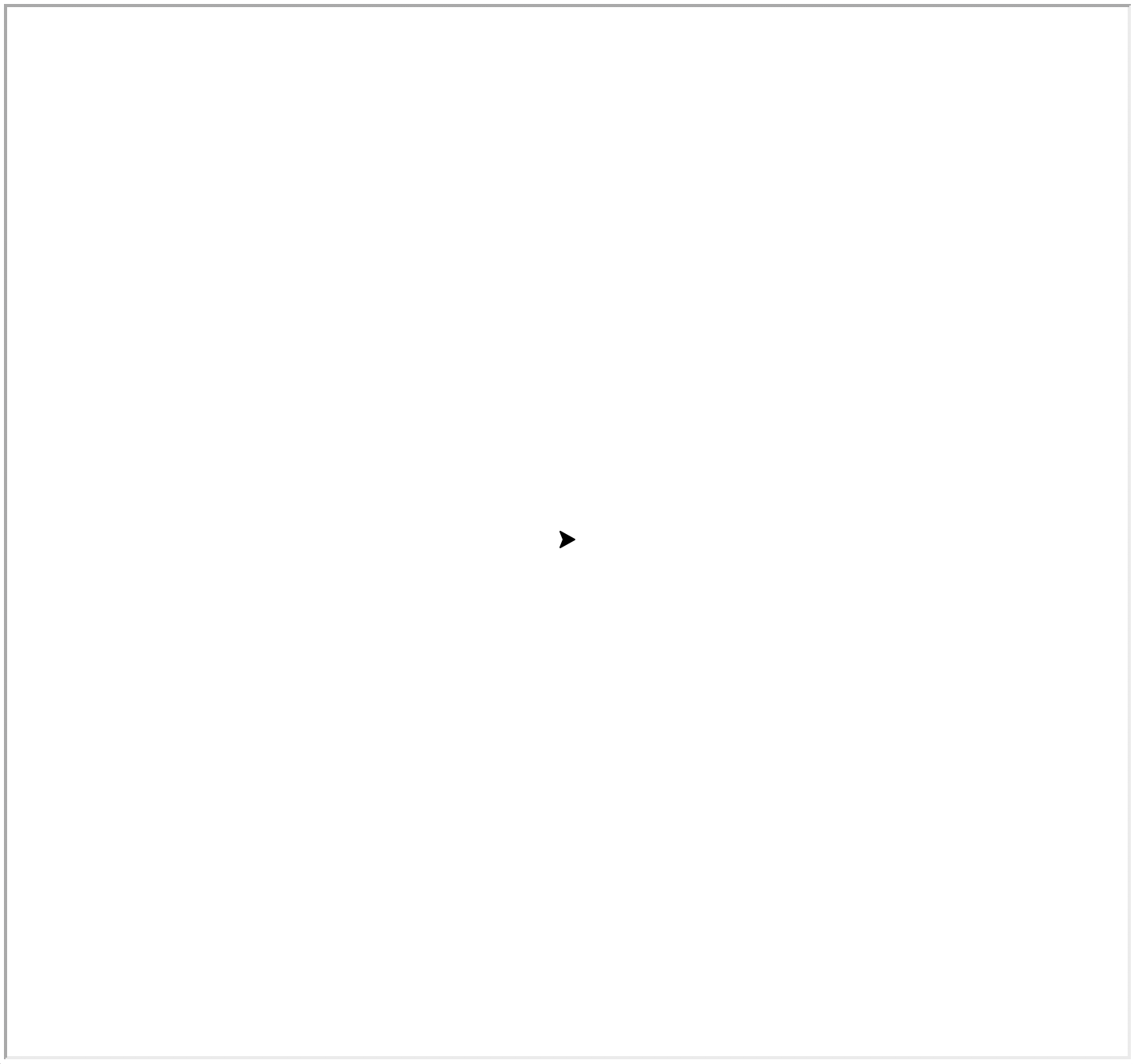We can now issue a command for the turtle to follow:

``````Python
>>> t.forward(100)``````

Here is the result: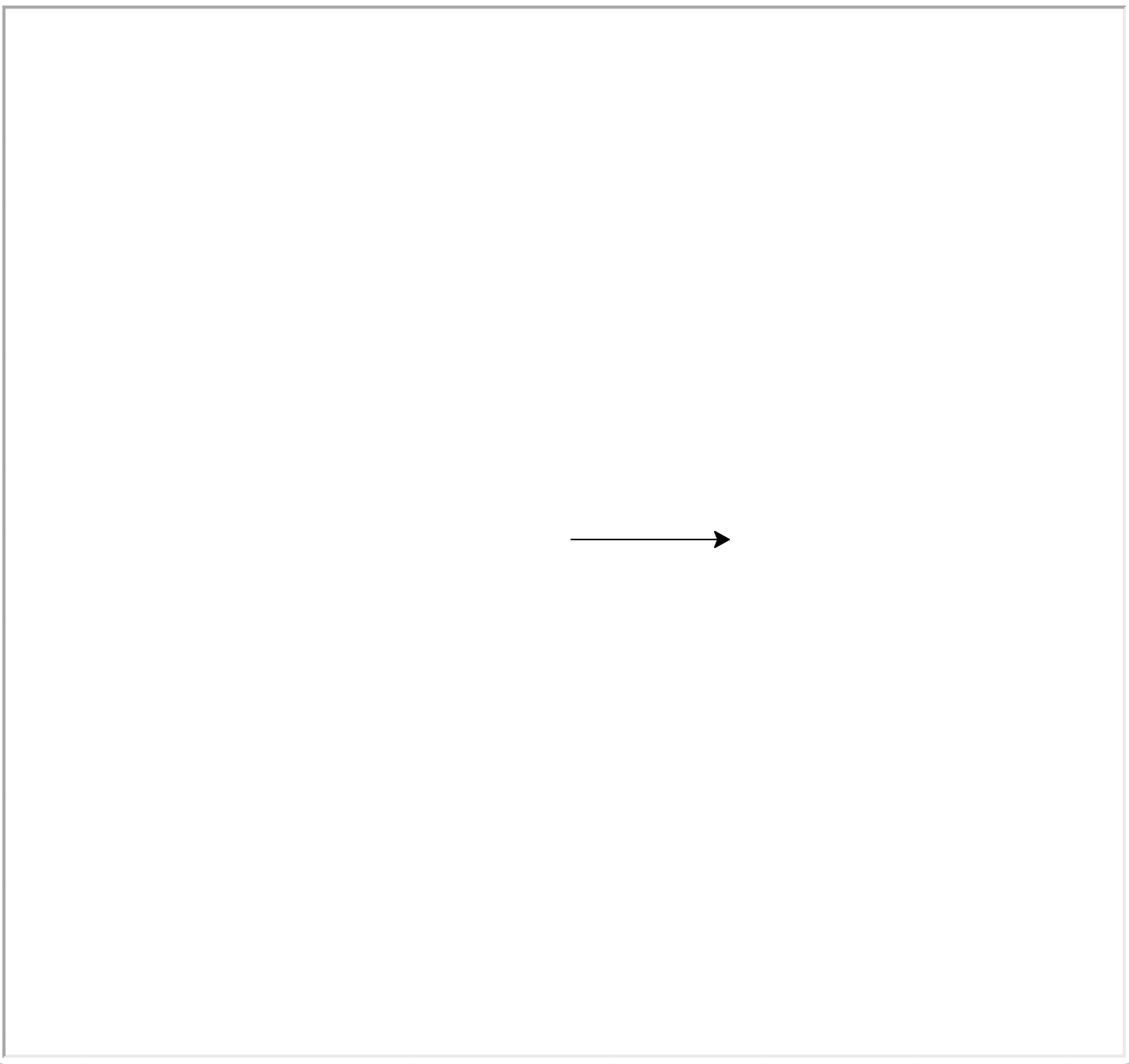We can complete the square by turning repeatedly by ninety degrees and moving forward.

``````Python
>>> t.right(90)
>>> t.forward(100)
>>> t.right(90)
>>> t.forward(100)
>>> t.right(90)
>>> t.forward(100)``````

The final result is a square of side 100, with the turtle in its original position, but pointing upwards: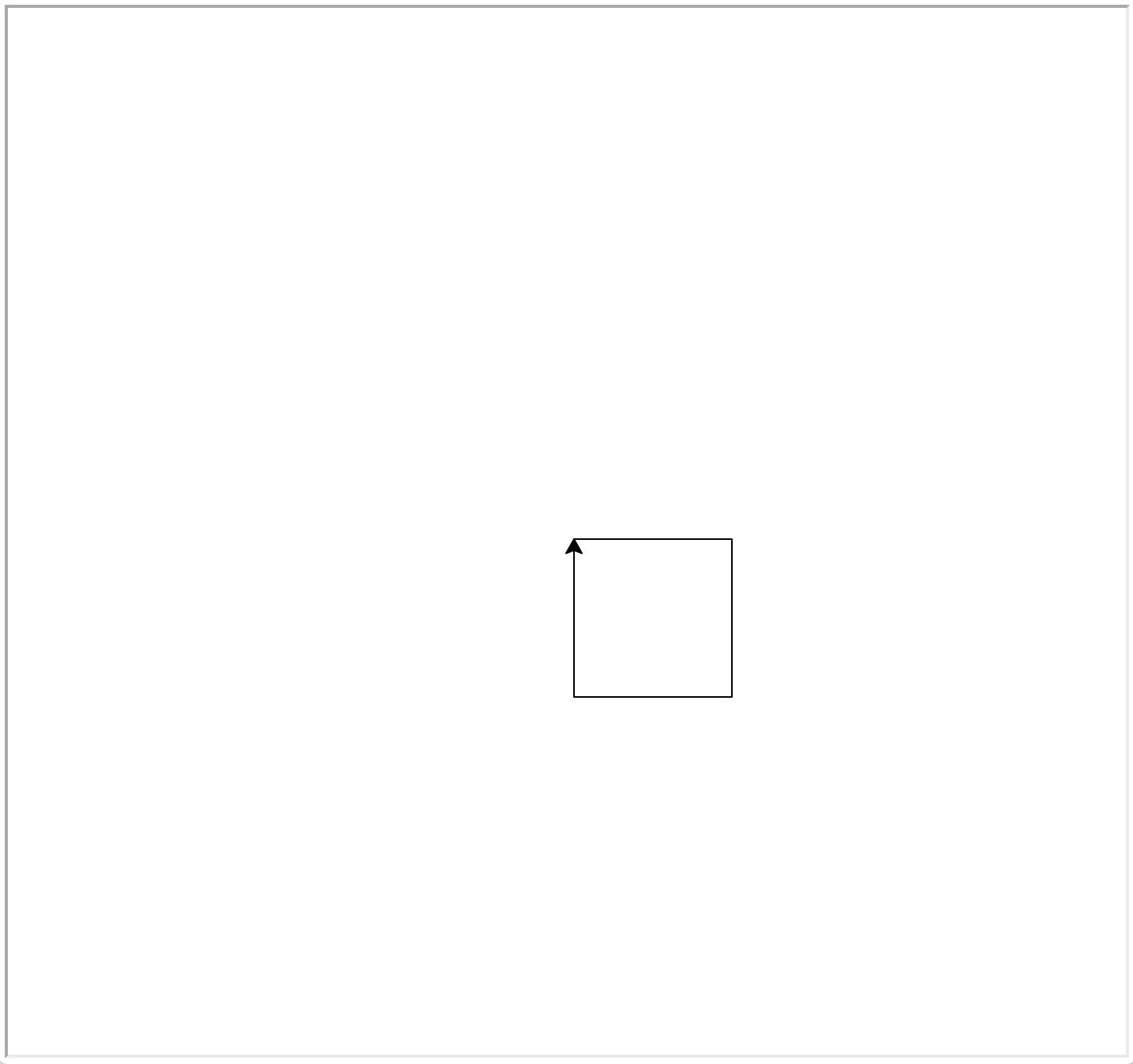We can write a function to make a square of any size:

``````Python
>>> def square(x):
...     for _ in range(4):
...         t.fd(x)
...         t.rt(90)``````

The functions `fd` and `rt` are abbreviations for `forward` and `right`. The underscore `_` is used to indicate that we are not using the counter from the `for` loop. We can make a primitive star by using `square` multiple times:

``````Python
>>> for _ in range(10):
...     square(100)
...     t.rt(360/10)``````

Here is the result: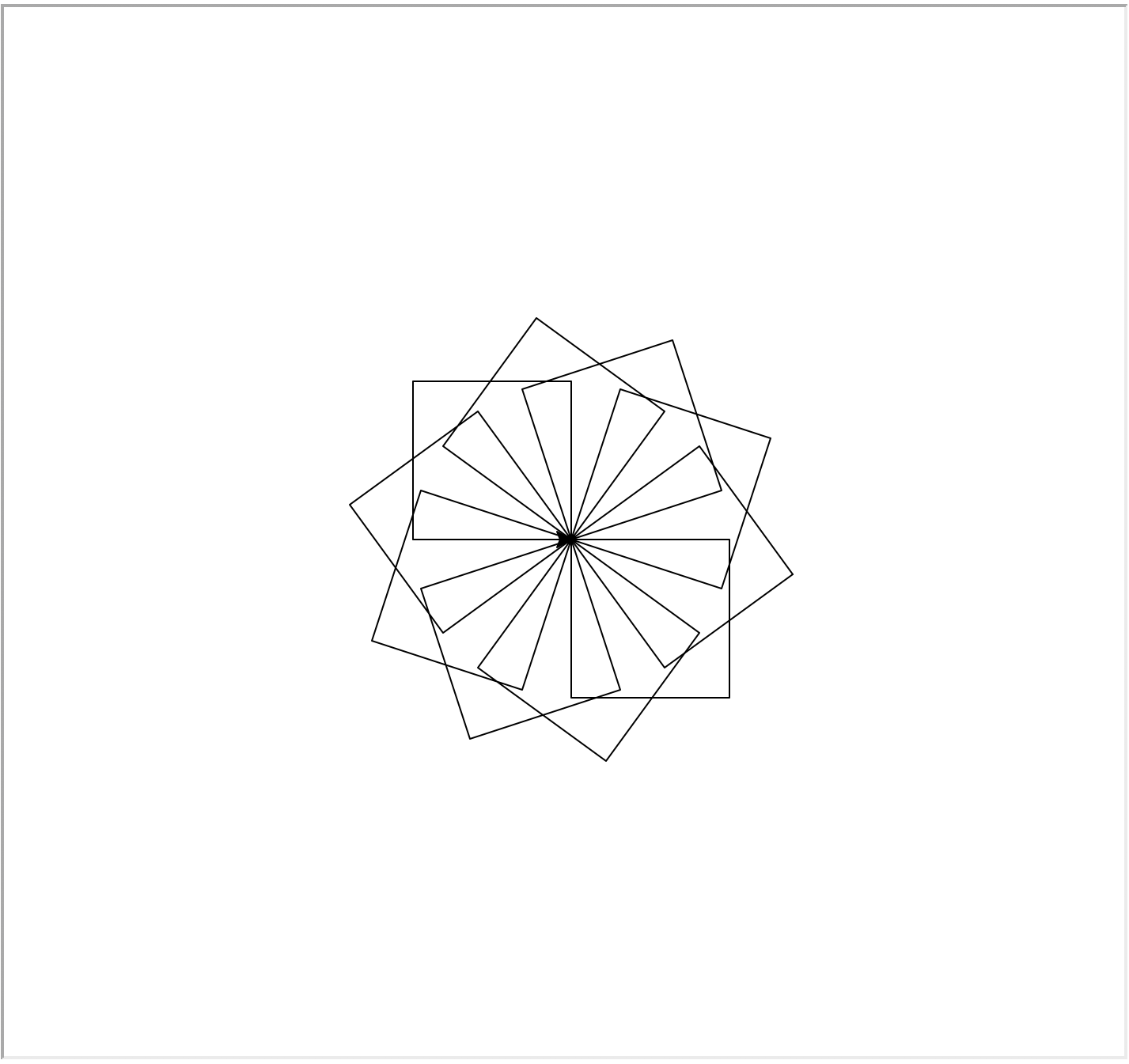When experimenting, the methods `home` and `clear` are useful:

``````Python
>>> t.home()
>>> t.clear()``````

The `home` method moves the turtle to the origin and restores its direction to the default. The `clear` function clears the turtle screen.

Question 1 Write a function `many_squares` which, given a number of squares to use and a size for the ‘star‘, draws it.

To make another kind of star, we can use the `backward/bk` method: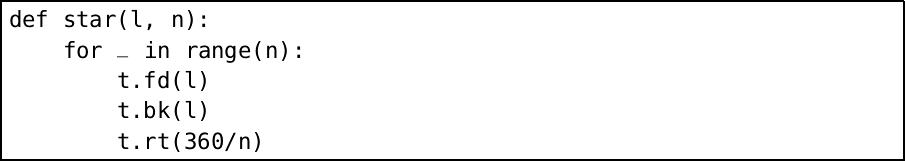Here is `star(100, 20)`: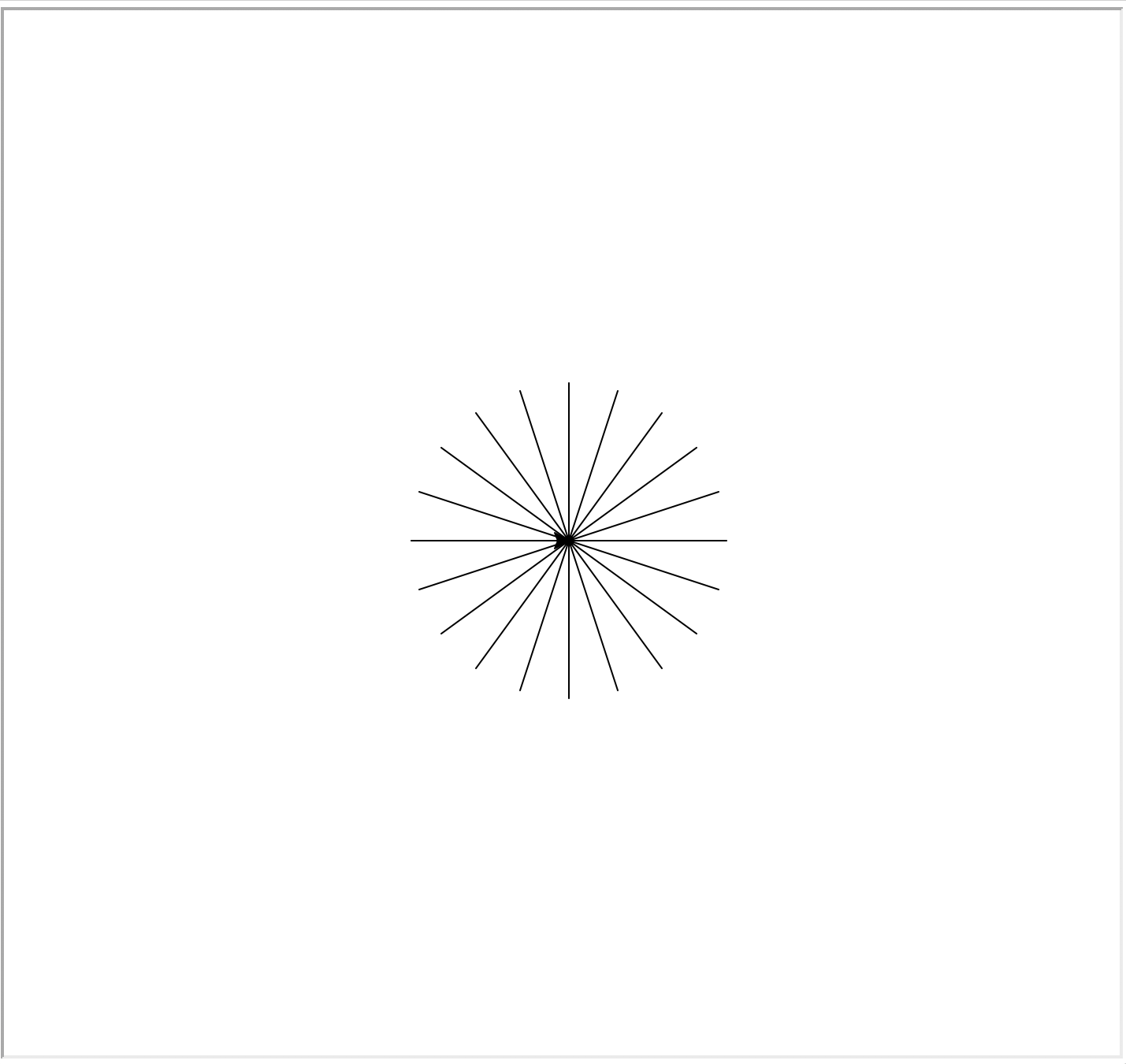There is a `left/lt` equivalent to `right/rt` as well.

Question 2 Write a function to draw a polygon with a given number of sides of a given length. Use this function, together with right turns, to repeat the given polygon multiple times to make a symmetrical pattern.

Question 3 Write a function `circle`, which draws a circle for a given centre position and radius, choosing the number of sides dependent on size to give a smooth result. If you need π, it can be found as `math.pi` after using `import`` math`.

So far we have no way to prevent the turtle leaving a trail behind. What if we want to draw multiple stars? We can use the methods `penup` (stop drawing a trail) and `pendown` (resume drawing a trail):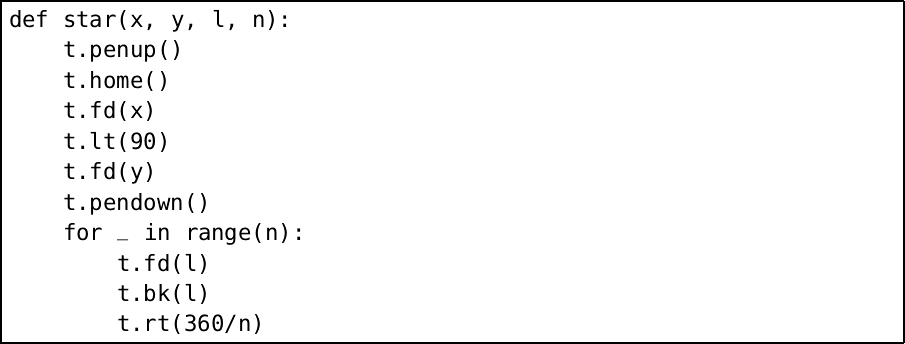Now we can use the `random` module to draw lots of stars:

``````Python
>>>import random
>>>for _ in range(20):
...    star(random.randint(-300, 300),
...         random.randint(-300, 300),
...         random.randint(10, 150),
...         random.randint(3, 30))``````

Here is the result of one run: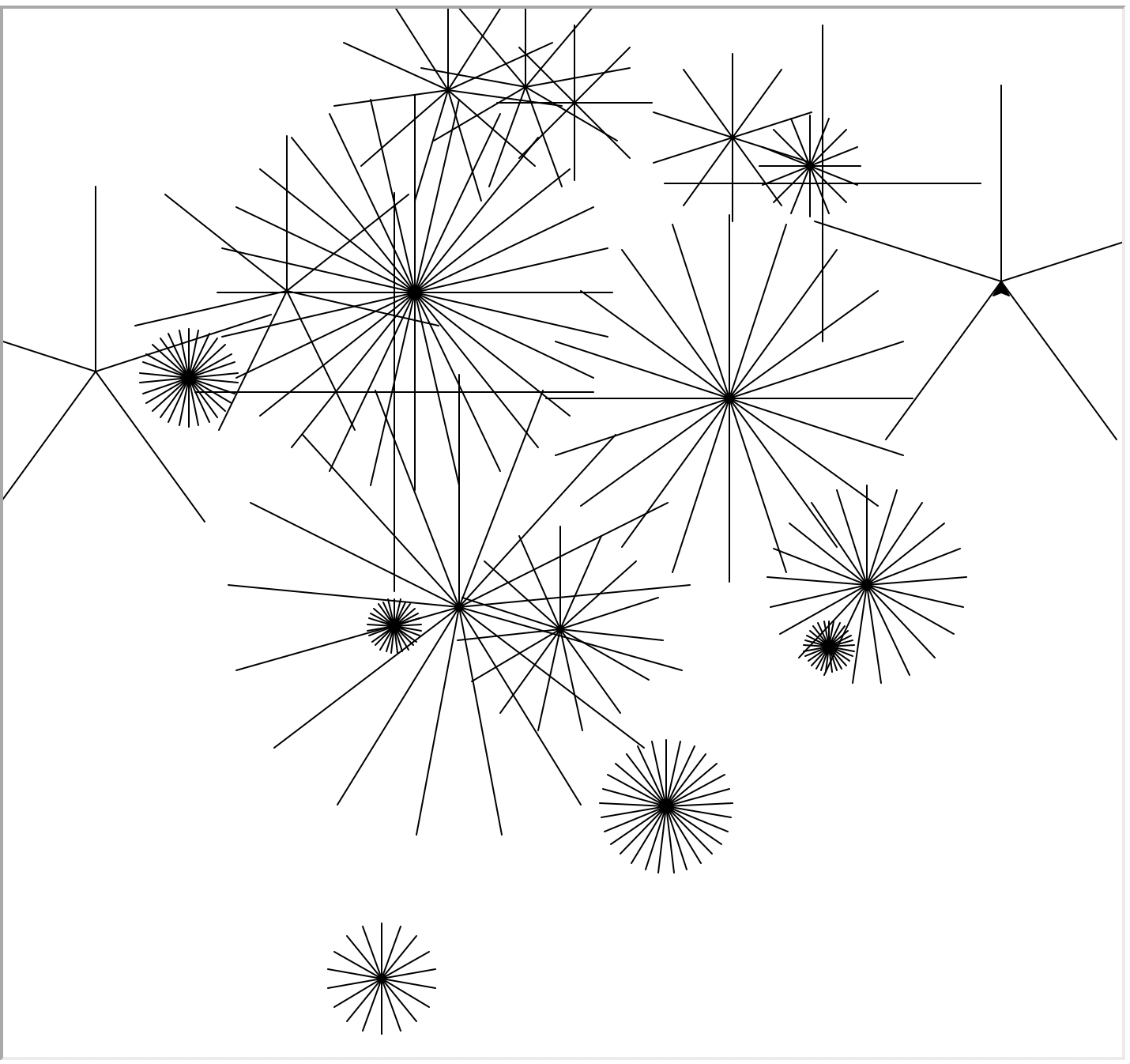We can simplify by using the method `goto` which moves to a given coordinate directly. We also use `setheading` to start each star at a random angle: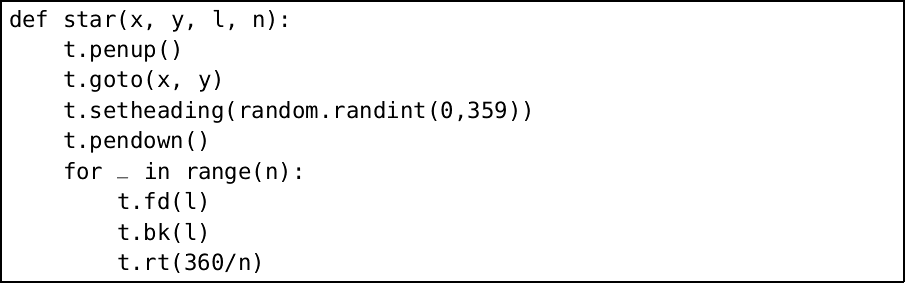Question 4 Using the `goto` method, write a function to draw a square grid of circles of diameter fifty which touch one another.

There are two problems with our pictures: they take a long time to draw, and the turtle gets in the way of the final result. To improve the speed, we use the `speed` method, which takes a number from 1 (slowest) to 10 (fastest). In addition, the number 0 means that no animation takes place, and the picture is drawn as quickly as possible. We can stop the turtle getting in the way of our final picture by using the `hideturtle` method (it has an opposite in `showturtle`). Try this:

``````Python
>>> t.hideturtle()
>>> t.speed(0)
>>> star(0, 0, 200, 7)
>>> t.showturtle()``````

The method `pensize` can be used to change the thickness of the trail. The `pencolor` method may be used to change the colour. The default pen width is 1 and, as we know, the default pen colour is black, which is the same as the red-green-blue triple (0, 0, 0). Consider this sequence of commands, where we use various shades of grey from black (0, 0, 0) to white (1, 1, 1):

``````Python
>>> t.pensize(20)
>>> star(0, 0, 200, 7)
>>> t.pensize(15)
>>> t.color(0.25, 0.25, 0.25)
>>> star(0, 0, 200, 7)
>>> t.pensize(10)
>>> t.color(0.5, 0.5, 0.5)
>>> star(0, 0, 200, 7)
>>> t.pensize(5)
>>> t.color(0.75, 0.75, 0.75)
>>> star(0, 0, 200, 7)
>>> t.pensize(2)
>>> t.color(1, 1, 1)
>>> star(0, 0, 200, 7)
>>> t.hideturtle()``````

Here is the result: